# Or, a tale in which one nerd spends far too long picking a Color for his blog with journeys into some fun bits of hexadecimal numbers, math, and programming. #

## Joining Svbtle #

I recently joined the ranks of Svbtle with this blog.

I did so for many reasons, but here are the highlights:

• I’ve long been a big fan of Dustin Curtis and his design chops
• I got tired of maintaining my own blog
• My old blog was in Jekyll which made editing it on a smartphone a no go
• I find the design clean and brilliant
• I tried a handful of others and this was my clear favorite

Thankfully, Svbtle takes care of most things for me, with choices I would have made myself.

The one main decision left to me for the look and feel was my Accent Color and I wanted to choose a great, unique one.

## Vibrant Colors #

Anyone who knows me well knows I’m a huge fan of vibrant colors. I ride a bright yellow motorcycle, the bright orange walls of SocialRadar, my employer fill me with joy, and so on.

I’m a firm believer that just about every color, in the right context, can be beautiful but my faves are bright ones that pop.

So picking just one accent color would be tricky, I just knew I wanted it bright and vibrant.

## A Light RGB Primer #

In the RGB color scheme used on the web, colors are denoted by 3 hexadecimal numbers with 2 digits each. One each for red, green, and blue.

Each hexadecimal number is 1-byte and thus has a range of (in decimal) zero to 256 shades. In hexadecimal this is 00-FF.

So a full RGB color hex triplet as used on the web is created by taking these three 2-digit hexadecimal numbers and jamming them together. This results in a sequence of the form #RRGGBB where each set of 2 hexadecimal numbers combine to form each color. The first 2 red, the second 2 green, the third 2 blue and together they describe a color.

So a simple color that includes all red and no green or blue would look like:

``````#FF0000
``````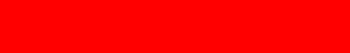That’s full red (FF hex, 256 decimal), empty green (00 hex, 0 decimal) and empty blue (00 hex, 0 decimal). A color that is all green and no red or blue would look like:

``````#00FF00
``````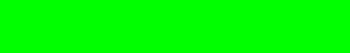That’s empty red (00 hex, 0 decimal), full green (FF hex, 256 decimal) and empty blue (00 hex, 0 decimal). Just to round it out:

``````#0000FF
``````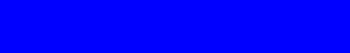That’s empty red, empty green, full throttle on the blue.

But of course the interesting bits lie in the combination of reds, greens, and blues in varying proportions. Most colors are not just one of these 3 colors full blast all by itself, but rather nice blends.

For example, the shade used by the DC Metro map for the Orange Line with a nice blend of all three:

``````#F4912C
``````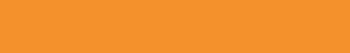Since there are three hex numbers which can range from 0 - 256 (in decimal) for each color, this means there are 256×256×256 total color combinations in the standard web RGB space, or 16,777,216 different colors.

That’s a lot to choose from!

I needed a starting point, some inspirado.

## The Golden Ratio #

I’ve been long fascinated by the Golden Ratio and its application to nicely proportional design.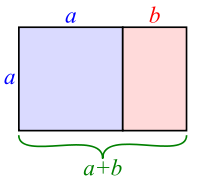image courtesy of wikipedia

Some suggest the Parthenon was built using the Golden Ratio for its beautiful proportions. 1

The idea that there is a mathematical formula which can render proportions that our brains find naturally appealing is a very pleasant one to me. A nice crossover between science and art.

So I started with the Golden Ratio as my inspirado, but its primary use is proportionality in spatial design, not in colors or numerics.

## Enter the Fibonacci sequence #

The Golden Ratio has an entangled lover known as the Fibonacci sequence.

In the simplest terms, the Fibonacci sequence is a series of numbers, in which each number is equal to the sum of the previous 2. Starting with `0` and `1`, the Fibonacci series looks as follows:

``````0, 1, 1, 2, 3, 5, 8, 13, 21, 34, 55, 89, 144, 233, 377, 610, ...
``````

Because `0 + 1 = 1`, `1 + 1 = 2`, `1 + 2 = 3`, `2 + 3 = 5`, `3 + 5 = 8`, and so on.

The interesting bit about the Fibonacci sequence is that, as the numbers get very large, the ratio between each number to its predecessor approaches the Golden Ratio.

For example, if we take the following snippet of JavaScript (with an assist from lodash), we can find the ratio between the nth Fibonacci number and its predecessor:

``````var _ = require('lodash');

// Helper function for generating the nth Fibonacci number
var fibonacci = _.memoize(function(n) {
return n < 2 ? n : fibonacci(n - 1) + fibonacci(n - 2);
});

var fibonacci_ratio = function(n) {
return fibonacci(n) / fibonacci(n - 1);
};

_.times(20, function(n) {
console.log(fibonacci_ratio(n));
});
``````

Using the above JavaScript (you can run it in Node.js), we end up with the following output:

``````0
Infinity
1
2
1.5
1.6666666666666667
1.6
1.625
1.6153846153846154
1.619047619047619
1.6176470588235294
1.6181818181818182
1.6179775280898876
1.6180555555555556
1.6180257510729614
1.6180371352785146
1.618032786885246
1.618034447821682
1.6180338134001253
1.618034055727554
``````

See how quickly the ratio of one Fibonacci number to the previous approaches a stable number? That’s the golden ratio! Beautiful.

## Fibonacci-based colors #

Ok, so what the heck does this all have to do with colors?

I first started looking at colors which were made up of Fibonacci numbers, converted to hex. For example, my first attempt was:

``````#5990E9
``````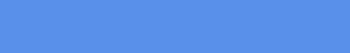That’s made up of 89 Red, 144 Green, 233 Blue, each is a Fibonacci number meaning 233 ÷ 144 is close to the Golden Ratio as is 144 ÷ 89.

Ok, so I dig that the ratios between the colors are like that, but that color just didn’t pop to me. It’s not quite vibrant enough for my tastes.

So let’s play a bit. If this color seemed washed out, so would any other color playing by the same scheme. So I decided purely Fibonacci proportional colors were out. It is my color after all so I can decide to move on ;)

## Variations on a theme #

Then I had an idea. While less pure but still fun, I was looking at the Fibonacci series and thinking, what if I jam them together and try it in hex to see how it looks. My first attempt:

``````#011235
``````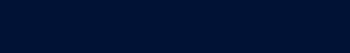Whoa, way too dark! But it is at least bold and not washed out like the purely Fibonacci color above. Let’s try taking it up one notch in brightness by skipping the leading `0`.

``````#112358
``````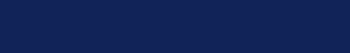Still too dark. Let’s try to shift once more. But wait, we can’t because the next Fibonacci number is `13` so it’s 2 digits. Oh but wait, we’re working in hexadecimal, so it’s not, that’s just `D`!

``````#12358D
``````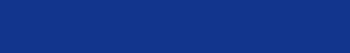Ok, now we’re getting somewhere, let’s try that shift one more time as we’re getting closer but not enough pop yet.

But the next Fibonacci number, `21`, would overflow as well. So let’s do the same trick as before. `21` in hex is `15`. Let’s drop the less significant digit there and give that a go:

``````#2358D1
``````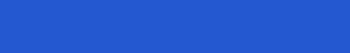Well that’s damned near perfection if you ask me. It’s a beautiful shade of blue, it’s vibrant, it pops, I think we have a winner!

So this is my Svbtle accent color. #2358D1. I’m staking my digital claim on that color!